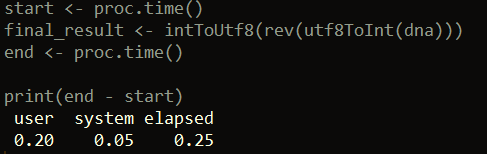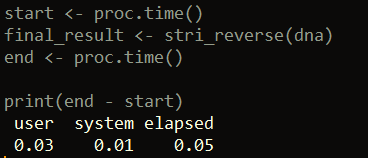Want to share your content on R-bloggers? click here if you have a blog, or here if you don't.R offers several ways to reverse a string, include some base R options. We go through a few of those in this post. We’ll also compare the computational time for each method.

Reversing a string can be especially useful in bioinformatics (e.g. finding the reverse compliment of a DNA strand). To get started, let’s generate a random string of 10 million DNA bases (we can do this with the stringi package as well, but for our purposes here, let’s just use base R functions).

```set.seed(1)
dna <- paste(sample(c("A", "T", "C", "G"), 10000000, replace = T), collapse = "")

```

## 1) Base R with strsplit and paste

One way to reverse a string is to use strsplit with paste. This is the slowest method that will be shown, but it does get the job done without needing any packages. In this example, we use strsplit to break the string into a vector of its individual characters. We then reverse this vector using rev. Finally, we concatenate the vector of characters into a string using paste.

```start <- proc.time()
splits <- strsplit(dna, "")[]
reversed <- rev(splits)
final_result <- paste(reversed, collapse = "")
end <- proc.time()

print(end - start)

```## 2) Base R: Using utf8 magic

This example also does not require any external packages. In this method, we can use the built-in R function utf8ToInt to convert our DNA string to a vector of integers. We then reverse this vector with the rev function. Lastly, we convert this reversed vector of integers back to its original encoding – except now the string is in reverse.

```start <- proc.time()
final_result <- intToUtf8(rev(utf8ToInt(dna)))
end <- proc.time()

print(end - start)

```## 3) The stringi package

Of all the examples presented, this option is the fastest when tested. Here we use the stri_reverse function from the stringi package.

```library(stringi)

start <- proc.time()
final_result <- stri_reverse(dna)
end <- proc.time()

print(end - start)

```## 4) The Biostrings package

Our last example uses the Biostrings package, which contains a collection of functions useful for working with DNA-string data. One function, called str_rev, can reverse strings. You can download and load the Biostrings package like this:

```source("http://bioconductor.org/biocLite.R")
biocLite("Biostrings")

library(Biostrings)

```

Then, all we have to do is input our DNA string into the str_rev function and we get our result.

```start <- proc.time()
final_result <- str_rev(dna)
end <- proc.time()

print(end - start)

```That’s it for this post! Please check out my other articles here.

The post Four ways to reverse a string in R appeared first on Open Source Automation.